# Subtracting decimals#### All in One Place

Everything you need for better grades in university, high school and elementary.#### Learn with Ease

Made in Canada with help for all provincial curriculums, so you can study in confidence.#### Instant and Unlimited Help

0/1
##### Intros
###### Lessons
1. Introduction to Subtracting Decimals:
How to subtract decimals with base ten (block) models
0/15
##### Examples
###### Lessons
1. Exchanging practice for decimals with block models
Practice exchanging to make more smaller place values. Use base ten (block) models to help.
1. 4 ones + 6 tenths = ___ ones + ___ tenths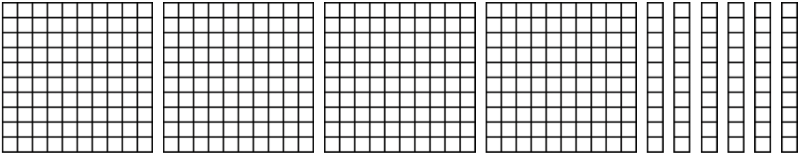2. Exchange 1 one for 10 tenths:
8 tens + 0 ones + 3 tenths = ___ tens + ___ ones + ___ tenths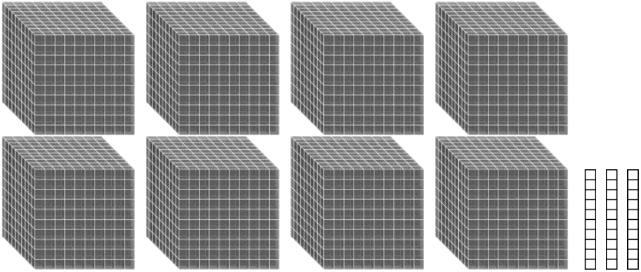3. Exchange 1 tenth for 10 hundredths:
9 tenths + 1 hundredth = ___ tenths + ___ hundredths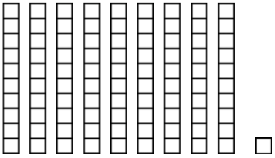4. Exchange 1 tenth for 10 hundredths:
2 ones + 0 tenths + 7 hundredths = ___ ones + ___ tenths + ___ hundredths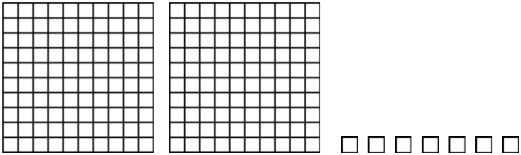2. Subtracting decimal block models
Subtract the decimal block models. Give the answer in standard form.
1.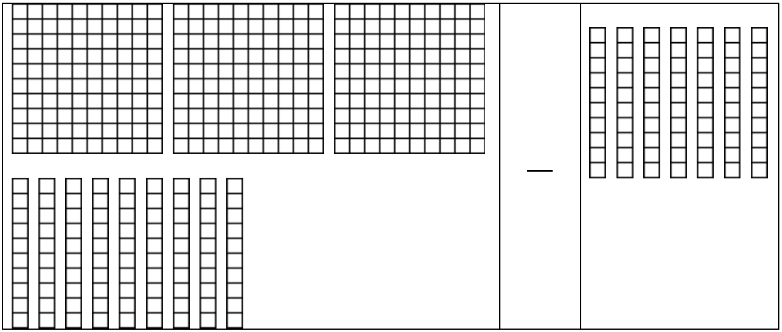2.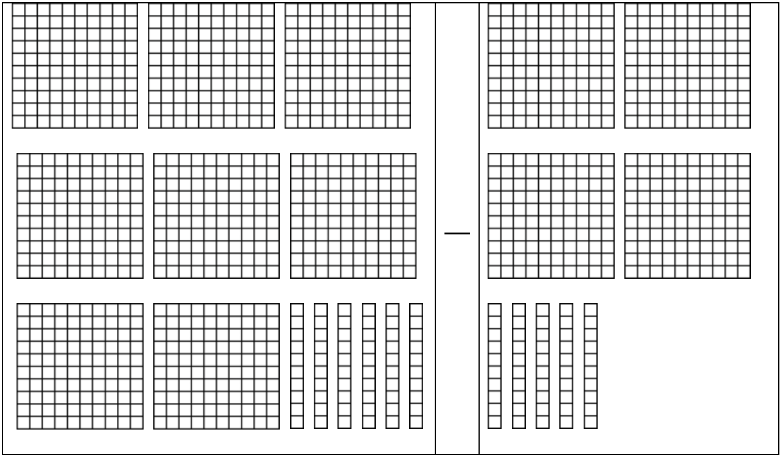3.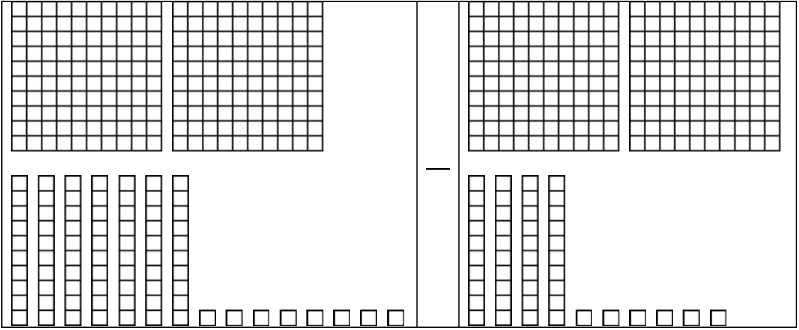4.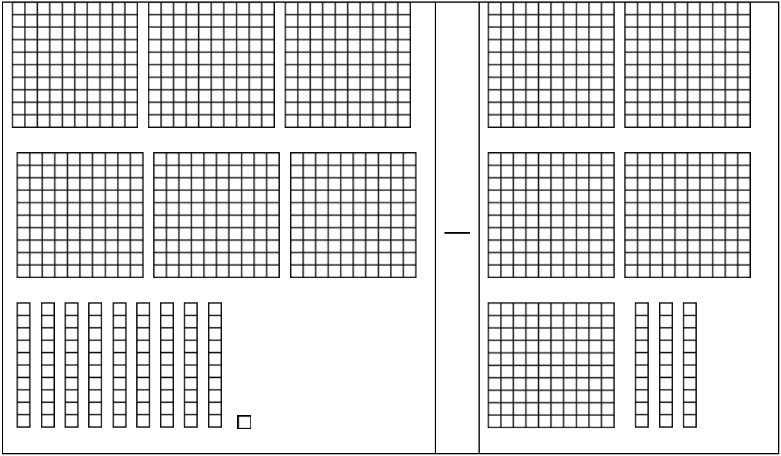3. Subtracting decimals on a grid
Subtract the decimals by lining up on the grid.
1. 18.7 - 2.3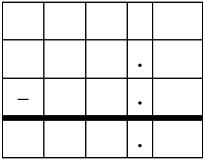2. 57.98 - 8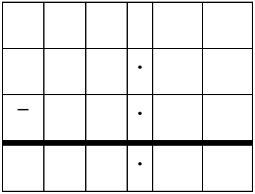3. 9.2 - 5.4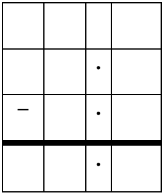4. 78.03 - 9.56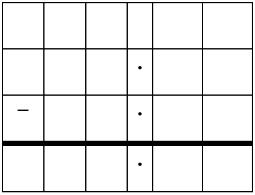4. Subtracting decimals and rounding decimal differences
1. 41.2 - 8.43
2. 62.04 - 31.03
3. 675.92 - 324.7
0%
##### Practice
###### Topic Notes

In this lesson, we will learn:

• How to subtract decimal numbers using base ten (block) models
• That subtracting decimal numbers is the same as subtracting whole numbers, which involves lining up the right place values and exchanging

Notes:

• To subtract decimals with base ten (block) models:
• Remember the three types of blocks used to represent the ones, tenths, and hundredths place values
• "One whole" or hundred block = ones place value
• Column (stick or rod) = tenths place value
• Single square = hundredths place value• A subtraction statement is made of: minuend - subtrahend = difference
• Cross out the blocks that you are subtracting (subtrahend from the minuend), until you've crossed out all matching pairs (subtrahend all crossed out).
• Exchange (or "borrow") from a bigger block if you don't have enough blocks to cross out. One big block can be split into 10 of the next smaller type of block (next place value).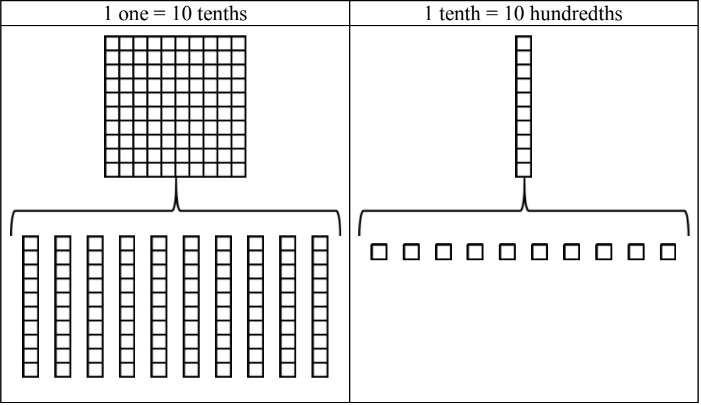• To subtract decimals, line up the decimal point to make sure you are adding the right place values in the same columns. Exchange (borrow) whenever necessary.
• Sometimes, you will have to write one or more extra zeroes to a number after the decimal point (trailing zeroes) when the minuend/subtrahend don't have the same number of place values.